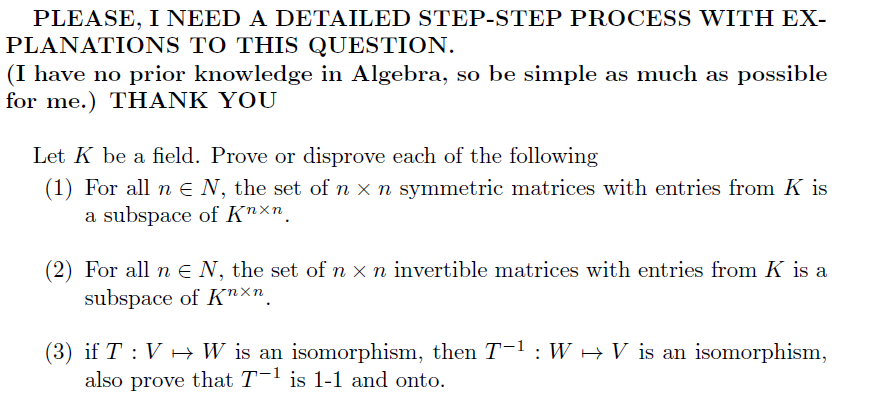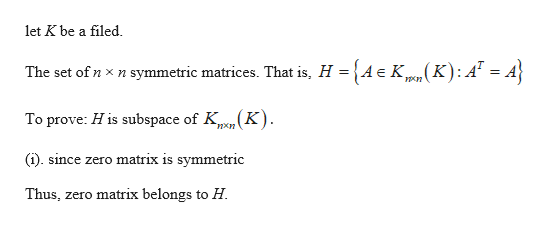# PLEASE, I NEED A DETAILED STEP-STEP PROCESS WITH EX-PLANATIONS TO THIS QUESTION.(I have no prior knowledge in Algebra, so be simple as much as possiblefor me.) THANK YOULet K be a field. Prove or disprove each of the following(1) For all ne N, the set of n x n symmetric matrices with entries from K isa subspace of Knxn(2) For all ne N, the set of n x n invertible matrices with entries from K is asubspace of Knxn(3) if T V W is an isomorphism, then T-1 : Walso prove that T1 is 1-1 and ontoV is an isomorphism

Question
46 views

I have no strong background in Algebra, so be simple and basic as much as possible for me to grasp each step that you will be provided. I will also rate your answer very high. Thank you so much.help_outlineImage TranscriptionclosePLEASE, I NEED A DETAILED STEP-STEP PROCESS WITH EX- PLANATIONS TO THIS QUESTION. (I have no prior knowledge in Algebra, so be simple as much as possible for me.) THANK YOU Let K be a field. Prove or disprove each of the following (1) For all ne N, the set of n x n symmetric matrices with entries from K is a subspace of Knxn (2) For all ne N, the set of n x n invertible matrices with entries from K is a subspace of Knxn (3) if T V W is an isomorphism, then T-1 : W also prove that T1 is 1-1 and onto V is an isomorphism fullscreen
check_circle

Step 1
1.

Note that, a subspace of a vector space V is a subset H of V that has three properties:

(i). The zero vectors of V is in H.

(ii). For each u and v are in H, u + v is in H.

(iii). For each u in H and each scalar c, cu is in H.help_outlineImage Transcriptioncloselet K be a filed The set of n x n symmetric matrices. That is, H = n K) To prove: H is subspace of K (). since zero matrix is symmetric Thus, zero matrix belongs to H fullscreen
Step 2

(ii).

Let  A and B belong to H.

Here, A and B are symmetric. That is,

Step 3

Check closed under ve...

### Want to see the full answer?

See Solution

#### Want to see this answer and more?

Solutions are written by subject experts who are available 24/7. Questions are typically answered within 1 hour.*

See Solution
*Response times may vary by subject and question.
Tagged in

### Other